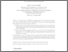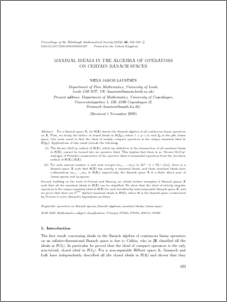# Maximal ideals in the algebra of operators on certain Banach spaces.

Laustsen, Niels J. (2002) Maximal ideals in the algebra of operators on certain Banach spaces. Proceedings of the Edinburgh Mathematical Society, 45 (3). pp. 523-546. ISSN 0013-0915Preview
For a Banach space $\mathfrak{X}$, let $\mathcal{B}(\mathfrak{X})$ denote the Banach algebra of all continuous linear operators on $\mathfrak{X}$. First, we study the lattice of closed ideals in $\mathcal{B}(\mathfrak{J}_p)$, where $1 < p < \infty$ and $\mathfrak{J}_p$ is the $p$th James space. Our main result is that the ideal of weakly compact operators is the unique maximal ideal in $\mathcal{B}(\mathfrak{J}_p)$. Applications of this result include the following. (i) The Brown–McCoy radical of $\mathcal{B}(\mathfrak{X})$, which by definition is the intersection of all maximal ideals in $\mathcal{B}(\mathfrak{X})$, cannot be turned into an operator ideal. This implies that there is no ‘Brown–McCoy’ analogue of Pietsch’s construction of the operator ideal of inessential operators from the Jacobson radical of $\mathcal{B}(\mathfrak{X})/\mathcal{A}(\mathfrak{X})$. (ii) For each natural number $n$ and each $n$-tuple $(m_1,\dots,m_n)$ in $\{k^2\mid k\in\mathbb{N}\}\cup\{\infty\}$, there is a Banach space $\mathfrak{X}$ such that $\mathcal{B}(\mathfrak{X})$ has exactly $n$ maximal ideals, and these maximal ideals have codimensions $m_1,\dots,m_n$ in $\mathcal{B}(\mathfrak{X})$, respectively; the Banach space $\mathfrak{X}$ is a finite direct sum of James spaces and $\ell_p$-spaces. Second, building on the work of Gowers and Maurey, we obtain further examples of Banach spaces $\mathfrak{X}$ such that all the maximal ideals in $\mathcal{B}(\mathfrak{X})$ can be classified. We show that the ideal of strictly singular operators is the unique maximal ideal in $\mathcal{B}(\mathfrak{X})$ for each hereditarily indecomposable Banach space $\mathfrak{X}$, and we prove that there are $2^{2^{\aleph_0}}$ distinct maximal ideals in $\mathcal{B}(\mathfrak{G})$, where $\mathfrak{G}$ is the Banach space constructed by Gowers to solve Banach’s hyperplane problem.View Item